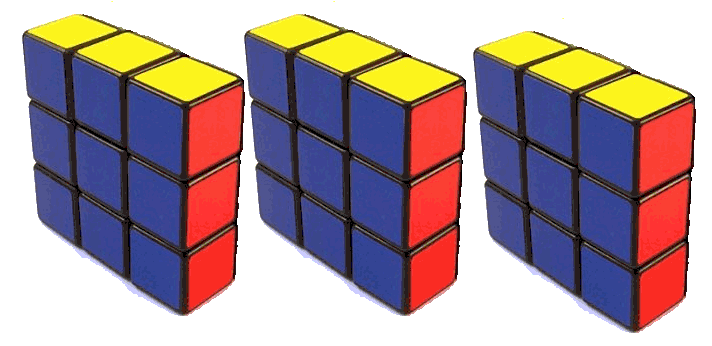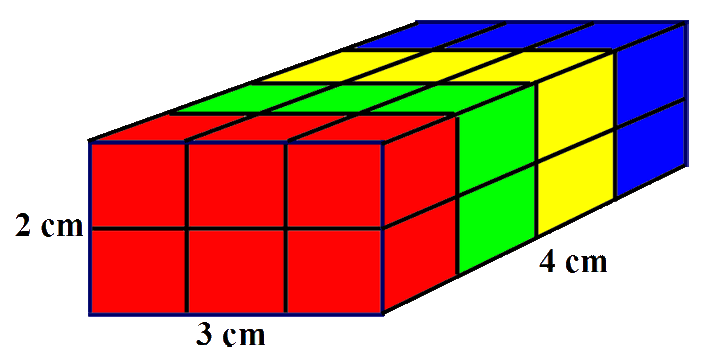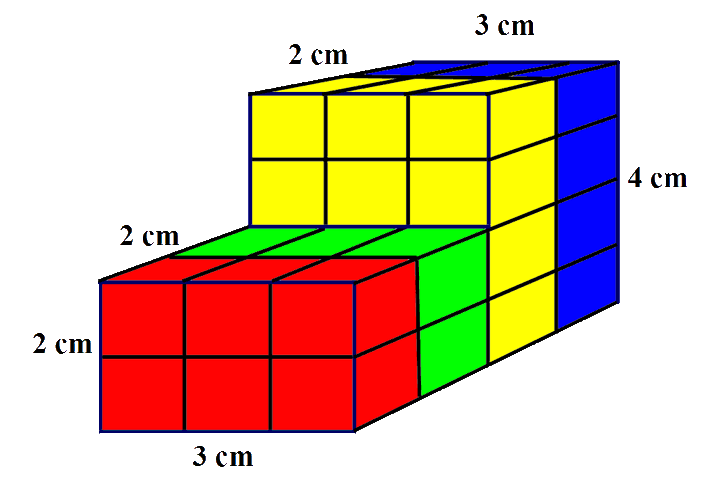# Shape and Space - Volume of 3D Shapes

## The Volume of a 3D shape is the space that the object takes up. It is the amount of space that the shape occupies.

### The units of volume are the cubes of the unit used to measure the shape. If the unit used is centimetres then the unit of measurement is cubic centimetres, cm³. If metres are used then the unit of measurement is cubic metres, m³.

This idea of cubic units can be difficult to understand - I explain it as the number of cubes of that size, 1 cm, that you would need to fill the object. Lets take an example of a cube that is 3cm wide, 3 cm high and 3cm deep - like a rubix cube (a small one with all edges 3cm).How many cubes that are 1 cm wide, 1 cm high and 1 cm deep would it take to fill the shape?

The best way is to draw it or make the shape - perhaps by using OXO cubes!In the diagram above, the rubix cube is split into 3 slices to help to visualise the shape.

You can see that it takes nine small cubes to make each slice, There are three slices -
so that is 3 x 9 = 27 small cubes to make up the whole cube.

So we can say that the volume of the shape is 27 centimetre cubes - we write this as, 27 cm³ .
We say this as "twenty seven cubic centimetres".

Clearly we cant do this for every shape that we wish to find the volume of. For a cuboid, all we need to do is count how many cubes would fit along each edge and then multiply them.In this case the width is 3 cm, the height is 2 cm and the depth is 4 cm. Now multiply them together - the volume is ... 3 x 2 x 4 = 24 cm³ or "24 cubic centimetres".

For more complex shapes, you may have to split the shape into two or more cuboids and work out the volume of each one individually and then combine the results.If we split the shape into 2 sections.

The red and green section is 3 cm wide, 2 cm high and 2 cm deep ... 3 x 2 x 2 = 12 cm³

The yellow and blue section is 3 cm wide, 4 cm high and 2 cm deep ... 3 x 4 x 2 = 24 cm³

Add the results ... 12 + 24 = 36 cm³

The total volume of the shape is 36 cm³

Practice working with Volume of 3D
shapes with these worksheets
from Primary Resources

Volume Visuals

lots of different shapes to work with.

Cubic Crazy

An investigation of cubes.